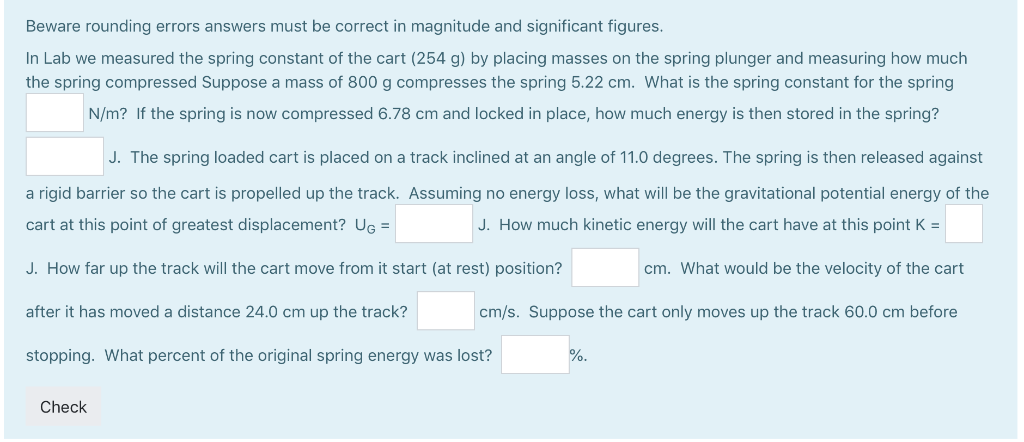# Beware rounding errors answers must be correct in magnitude and significant figures In Lab we mea...

###### Question:Beware rounding errors answers must be correct in magnitude and significant figures In Lab we measured the spring constant of the cart (254 g) by placing masses on the spring plunger and measuring how much the spring compressed Suppose a mass of 800 g compresses the spring 5.22 cm. What is the spring constant for the spring N/m? If the spring is now compressed 6.78 cm and locked in place, how much energy is then stored in the spring? J. The spring loaded cart is placed on a track inclined at an angle of 11.0 degrees. The spring is then released against a rigid barrier so the cart is propelled up the track. Assuming no energy loss, what will be the gravitational potential energy of the cart at this point of greatest displacement? UG J. How far up the track will the cart move from it start (at rest) position? after it has moved a distance 24.0 cm up the track? stopping. What percent of the original spring energy was lost? %. J. How much kinetic energy will the cart have at this point K- cm. What would be the velocity of the cart cm/s. Suppose the cart only moves up the track 60.0 cm before Check

##### How do you find the derivative of s(t)=t^3-2t+4?
(x^alpha)'=alpha*x^(alpha-1)...